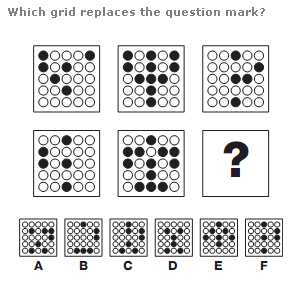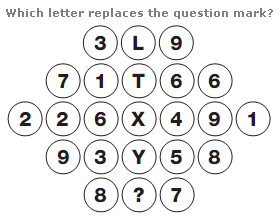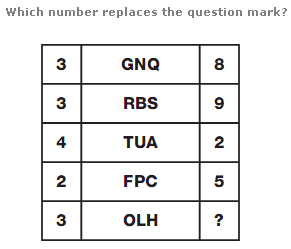# Puzzles - Logical puzzles

### Exercise :: Logical puzzlesAnswer : A Explanation : Working in rows, if you superimpose the pattern of spots in the left and right hand grids you get the pattern in the central grid.

View Answer & ExplanationAnswer : O Explanation : Working in rows, add up the digits in each row and put the letter with this numerical value in the centre of the row.

View Answer & ExplanationAnswer : 5 Explanation : In each row, add together the numerical values of the 3 central letters to give a 2 digit sum, and put this 2 digit sum in the left and right hand boxes at the end of the rows.

View Answer & Explanation

#### Current Affairs 2019

Interview Questions and Answers